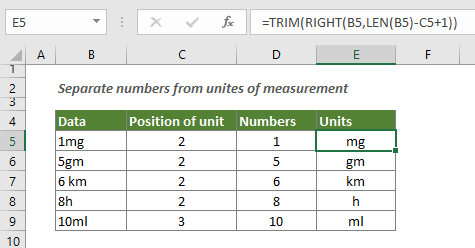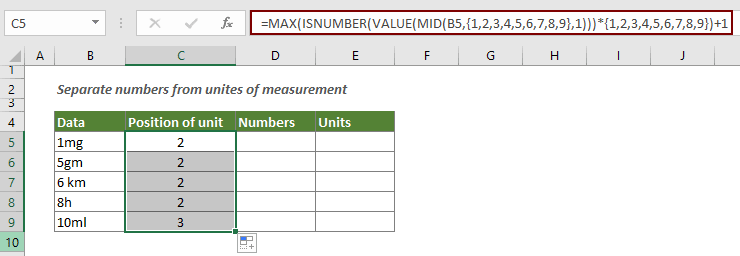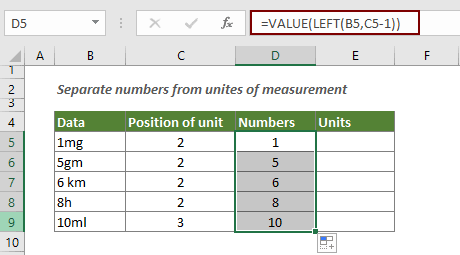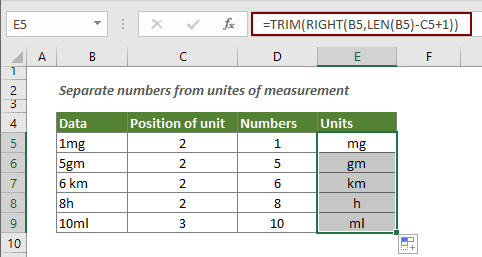## Separate numbers from units of measurement

This article explains how to use formula to separate numbers from unites of measurement.#### How to separate numbers from units of measurement?

The below three formulas can help you split numbers from units of measurement so that they are in two separate columns.

##### Firstly, locate the first character of unit

1. Select a blank cell to output the result.

2. Enter or copy the below formula into it and press the Enter key. Select the result cell and then drag the Fill Handle to apply the formula to other cells as you need.

=MAX(ISNUMBER(VALUE(MID(B5,{1,2,3,4,5,6,7,8,9},1)))*{1,2,3,4,5,6,7,8,9})+1##### Secondly, separate the numbers from units

1. Select a blank cell to output the result.

2. Enter the below formula into it and press the Enter key. Select the result cell and then drag the Fill Handle to apply the formula to other cells as you need.

=VALUE(LEFT(B5,C5-1))##### Finally, get the units

1. Select a blank cell to output the result.

2. Enter the below formula into it and press the Enter key. Select the result cell and then drag the Fill Handle to apply the formula to other cells as you need.

=TRIM(RIGHT(B5,LEN(B5)-C5+1))How these formulas work?

=MAX(ISNUMBER(VALUE(MID(B5,{1,2,3,4,5,6,7,8,9},1)))*{1,2,3,4,5,6,7,8,9})+1

• 1. MID(B5,{1,2,3,4,5,6,7,8,9},1): The MID function extracts the first 9 values in B5, and returns the result as an array: {"1","m","q","","","","","",""};
• VALUE({"1","m","q","","","","","",""}): The VALUE function converts numbers in text format to actual numbers and returns the result as {1,#VALUE!, #VALUE!, #VALUE!, #VALUE!, #VALUE!, #VALUE!, #VALUE!, #VALUE!};
• 2. ISNUMBER({1,#VALUE!, #VALUE!, #VALUE!, #VALUE!, #VALUE!, #VALUE!, #VALUE!, #VALUE!}) *{1,2,3,4,5,6,7,8,9}: The ISNUMBER function checks if there are numbers or not in the array and returns {TRUE,FALSE, FALSE, FALSE, FALSE, FALSE, FALSE, FALSE, FALSE}. And then this array multiplies another array with 9 numbers and returns {1,0,0,0,0,0,0,0,0,};
• 3. MAX({1,0,0,0,0,0,0,0,0,})+1: And then the MAX function get the largest value "1" in the array. This largest value is the position of the last number in B5. We need to add 1 to get the position of the first character of the unit. Here the result is 1+1=2.

=VALUE(LEFT(B5,C5-1)):

• The LEFT function extracts 1(2-1=1) character starting from the left side of B5 and returns 1. And then the VALUE function converts this number in text format to an actual number.

=TRIM(RIGHT(B5,LEN(B5)-C5+1))

• 1. LEN(B5): The LEN function calculates the total text length of B5. The result is 3;
• 2. TRIM(RIGHT(B5,2)): The RIGHT function extracts two characters starting from the right side of B5 and returns the result mg. And the TRIM function will remove all spaces from the result if there is space between number and unit.

Note: The first formula in this article only works well for the number which length is equal to or less than 9 characters.

#### Related functions

Excel MAX function
The Excel MAX function returns the largest value in a set of values.

Excel ISNUMBER function
The Excel ISNUMBER function checks whether a value is a number and returns TRUE or FALSE.

Excel VALUE function
The Excel VALUE function helps to convert a text string that represents a number (such as number, date or time format) into a number.

Excel MID function
The Excel MID function is used to find and return a specific number of characters from the middle of given text string.

Excel LEFT function
The LEFT function extracts the given number of characters from the left side of a supplied string.v

Excel TRIM function
The Excel TRIM function removes all extra spaces from text string and only keeps single spaces between words.

Excel RIGHT function
The RIGHT function extracts a specific number of characters from the right side of the text string.

#### Related formulas

Split dimensions into individual length, height and width
This article explains how to split dimensions in a cell into three parts (individual dimensions that include length, height and width).

Split dimensions into two parts in Excel
This article explains how to use formula to split dimensions in a cell into two parts without units (individual length and width).

### The Best Office Productivity Tools

#### Kutools for Excel - Helps You To Stand Out From Crowd

 Popular Features: Find, Highlight or Identify Duplicates  |  Delete Blank Rows  |  Combine Columns or Cells without Losing Data  |  Round without Formula ... Super VLookup: Multiple Criteria  |  Multiple Value  |  Across Multi-Sheets  |  Fuzzy Lookup... Adv. Drop-down List: Easy Drop Down List  |  Dependent Drop Down List  |  Multi-select Drop Down List... Column Manager: Add a Specific Number of Columns  |  Move Columns  |  Toggle Visibility Status of Hidden Columns  |  Compare Columns to Select Same & Different Cells ... Featured Features: Grid Focus  |  Design View  |  Big Formula Bar  |  Workbook & Sheet Manager | Resource Library (Auto Text)  |  Date Picker  |  Combine Worksheets  |  Encrypt/Decrypt Cells  |  Send Emails by List  |  Super Filter  |  Special Filter (filter bold/italic/strikethrough...) ... Top 15 Toolsets:  12 Text Tools (Add Text, Remove Characters ...)  |  50+ Chart Types (Gantt Chart ...)  |  40+ Practical Formulas (Calculate age based on birthday ...)  |  19 Insertion Tools (Insert QR Code, Insert Picture from Path ...)  |  12 Conversion Tools (Numbers to Words, Currency Conversion ...)  |  7 Merge & Split Tools (Advanced Combine Rows, Split Excel Cells ...)  |  ... and more

Kutools for Excel Boasts Over 300 Features, Ensuring That What You Need is Just A Click Away...#### Office Tab - Enable Tabbed Reading and Editing in Microsoft Office (include Excel)

• One second to switch between dozens of open documents!
• Reduce hundreds of mouse clicks for you every day, say goodbye to mouse hand.
• Increases your productivity by 50% when viewing and editing multiple documents.
• Brings Efficient Tabs to Office (include Excel), Just Like Chrome, Edge and Firefox.